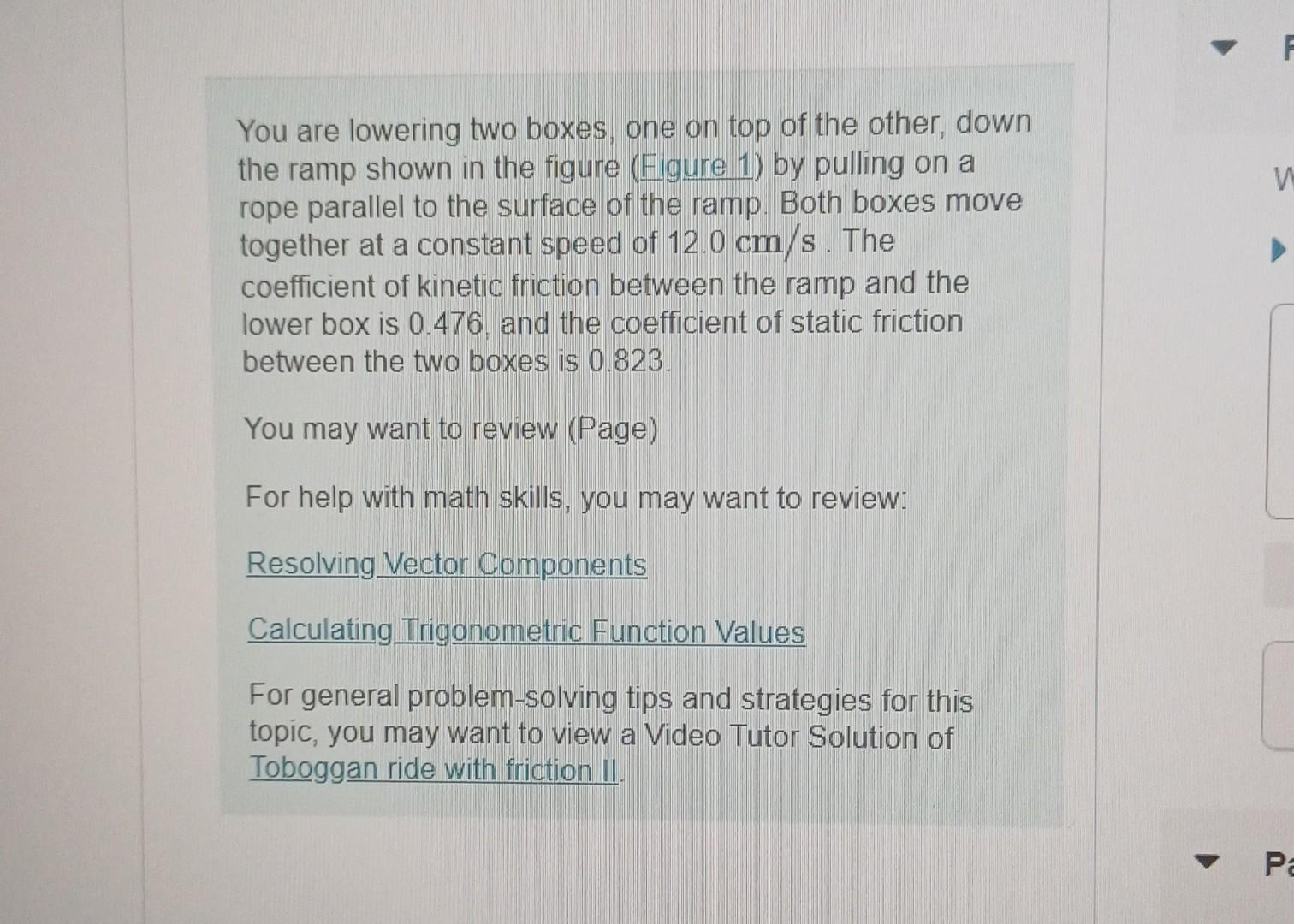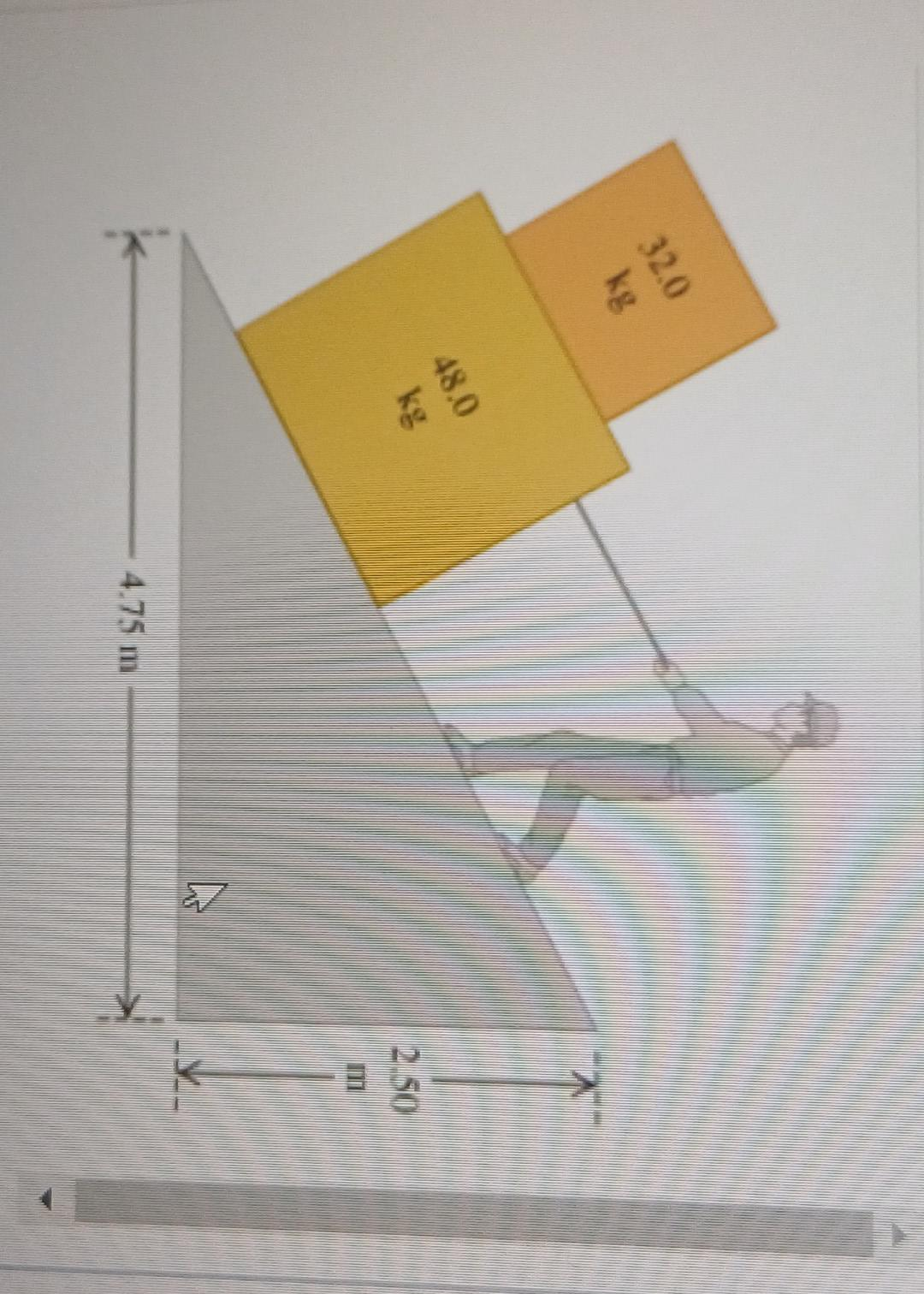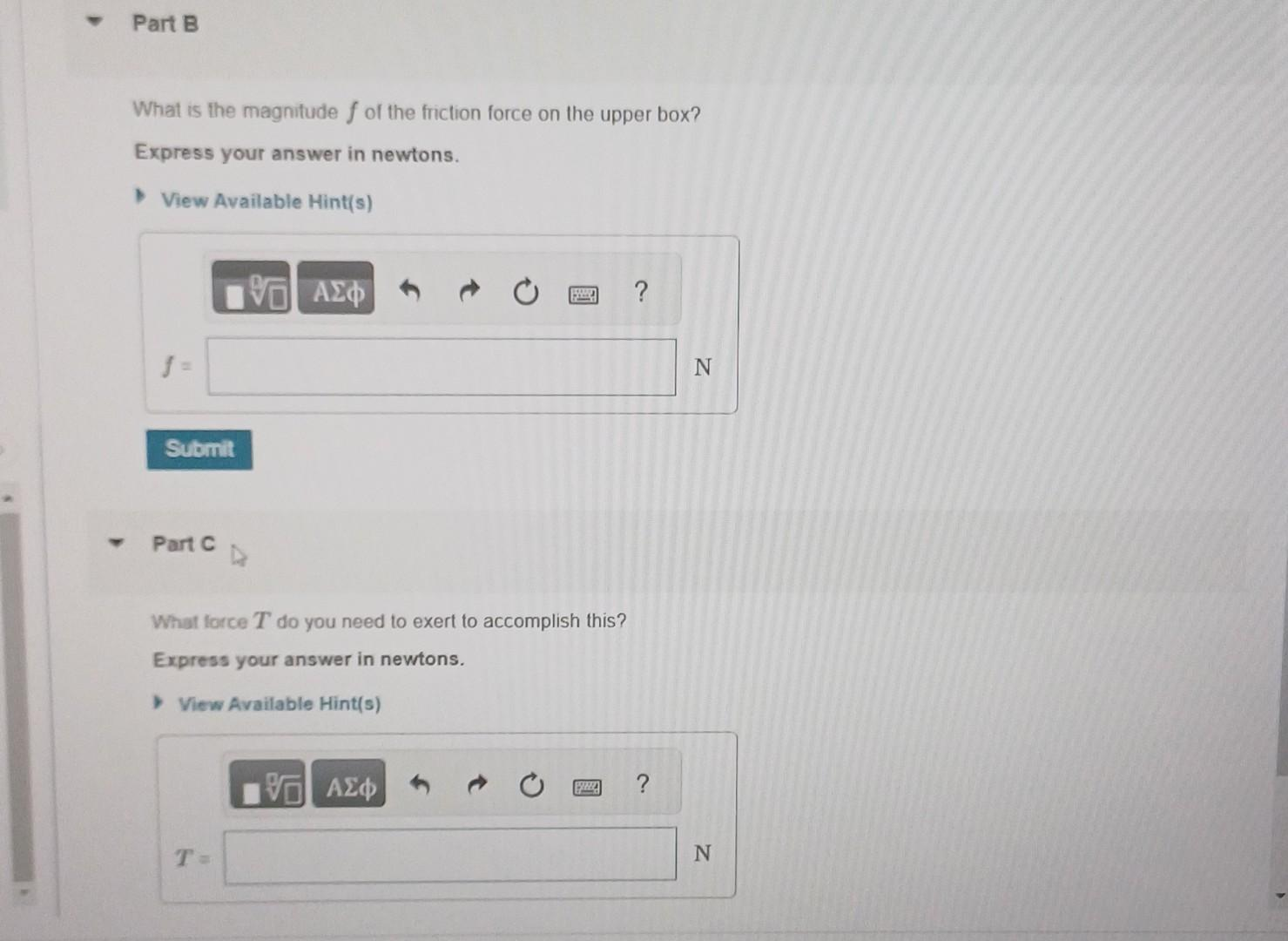Home / Expert Answers / Physics / you-are-lowering-two-boxes-one-on-top-of-the-other-down-the-ramp-shown-in-the-figure-pa642

# (Solved): You are lowering two boxes, one on top of the other, down the ramp shown in the figure ...You are lowering two boxes, one on top of the other, down the ramp shown in the figure (Figure 1) by pulling on a rope parallel to the surface of the ramp. Both boxes move together at a constant speed of . The coefficient of kinetic friction between the ramp and the lower box is 0.476 , and the coefficient of static friction between the two boxes is 0.823 . You may want to review (Page) For help with math skills, you may want to review: Resolving Vector Components Calculating Trigonometric Function Values For general problem-solving tips and strategies for this topic, you may want to view a Video Tutor Solution of Toboggan ride with friction II. What is the magnitude of the friction force on the upper box? Express your answer in newtons. Part C What lorce do you need to exert to accomplish this? Express your answer in newtons.

We have an Answer from Expert